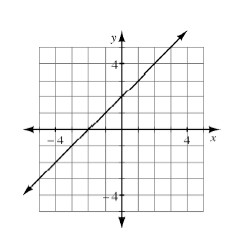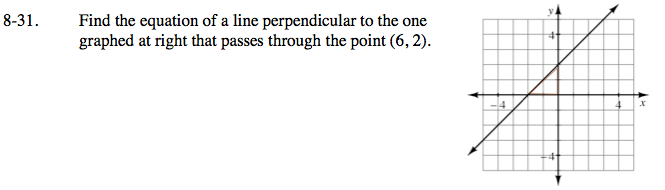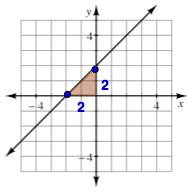### Home > CAAC > Chapter 8 > Lesson 8.1.3 > Problem8-31

8-31.

Find the equation of a line perpendicular to the one graphed below that passes through the point (6, 2). Homework Help ✎Find the slope of the line at right by making a slope triangle.

$\text{Slope}=\frac{2}{2}=1$Use the y = mx + b form of a line to start writing your equation.
Use the opposite reciprocal of the slope you found in order to make the line perpendicular to the one graphed at right.

y = −1x + b

Substitute the given point into the equation to solve for b.

2 = −1(6) + b
2 = −6 + b
8 = b

Substitute y and x back into their original places in the equation, and you have your equation for a line perpendicular to the one that is graphed at right.